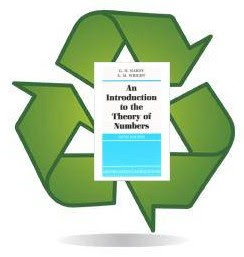## Saturday, 9 June 2012

### RECYCLING HARDY & WRIGHT

#### AVERAGE ORDER OF DEDEKIND PSI FUNCTION$latex \psi(n)= n \cdot \prod_{p|n}{\left( 1 + \frac{1}{p} \right)$

Dedekind psi function called my attention, when I was trying to find trivial properties and formulas related to Jordan's totient functions, that are indeed just generalizations of Euler's totient function. At that time I realize that values in range of psi had similarities to those of divisor sigma, because trivially: $latex \sigma_{1}(n) - \psi(n) \geq 0$, (inequality that holds for squarefree numbers). So, It seems reasonable to look for an alternate inequality to Robin's criterion but with Dedekind psi instead of divisor function.

Fortunately, this question was settled, before my discovery of gunpowder, by true mathematicians like Patrick Sole and Michel Planat ( and ).

Riemann Hypothesis (RH) is true if and only:

$latex \frac{\psi(n)}{n} < e^{\gamma} \;log{log{\;n}}$, for $latex n>30$.

After I found these papers on the internet, my interest on Dedekind psi function became huge, and then I begun to look for more and more information about this arithmetical function inside my library. But the real thing is that psi is never given the importance on every Number Theory book that it deserves. This was good to me because it gave me the opportunity to populate The On-Line Encyclopedia of Integer Sequences with my trivialities related to this function. This the modest mission of this blog: to create math not in the books.

As I'm a copycat and Euler's totient is first cousin of Dedekind psi, the idea is to turn $latex \varphi$ formulas into $latex \psi$ with a small retouch, for example if:

$latex \frac{n}{\varphi(n)}=\sum_{d|n}{\frac{{\mu(d)}^2}{\varphi(d)}}$

Then you can discover the unpublished:

$latex \frac{n}{\psi(n)}=\sum_{d|n}{\frac{\mu(d)}{\psi(d)}}$

The same thing can be done "recycling" the analytic theorems about the average order of number theoretical functions that came on the most cited book on the topic: Hardy and Wright's An Introduction to the Theory of Numbers, particularly Theorem 330 on the average order of Euler's Totient can be easily rewritten to get, the not so easy to find, average order of Dedekind psi, just implementing the following expressions along theorem development:

$latex \psi(n)= n \cdot \sum_{d|n}{ \frac{|\mu(d)|}{d}$

(Taken from )

and

$latex \sum_{d=1}^{\infty}{\frac{|\mu(d)|}{d^{2}}} = \frac{15}{{\pi}^{2}}$

(See )

Proof:
$latex \Psi(n) = \sum_{m=1}^{n}{\psi(m)} = m \cdot \sum_{d|m}{ \frac{|\mu(d)|}{d} =$

$latex \sum_{d \cdot d^{\prime} \leq n}{d^{\prime} \cdot |\mu(d)|} = \sum_{d=1}^{n}{|\mu(d)| \cdot \sum_{d^{\prime}=1}^{\lfloor \frac{n}{d} \rfloor}{d^{\prime}} =$

$latex \frac{1}{2} \sum_{d=1}^{n}{|\mu(d)| \bigg( \bigg\lfloor \frac{n}{d} \bigg\rfloor^{2} + \bigg\lfloor \frac{n}{d} \bigg\rfloor \bigg) =$

$latex \frac{1}{2} \sum_{d=1}^{n}{|\mu(d)| \bigg\{ \frac{n^{2}}{d^{2}} + O \bigg( \frac{n}{d} \bigg) \bigg\} } =$

$latex \frac{n^2}{2} \sum_{d=1}^{n}{ \frac{|\mu(d)|}{d^2} + O \bigg( n \sum_{d=1}^{n}{ \frac{1}{d} \bigg) } =$

$latex \frac{n^2}{2} \sum_{d=1}^{n}{ \frac{|\mu(d)|}{d^2} + O \bigg( n^{2} \sum_{n+1}^{\infty}{ \frac{1}{d^2} \bigg) + O(n \cdot \log{n}) =$

$latex \frac{15n^{2}}{2{\pi}^2} + O(n) + O(n \cdot \log{n}) = \frac{15n^{2}}{2{\pi}^2} + O(n \cdot \log{n})$

$latex \Psi(n) = \frac{15n^{2}}{2{\pi}^2} + O(n \cdot \log{n})$

Then the average order of $latex \psi(n)$ is $latex \frac{2}{n} \cdot \frac{15n^2}{{2\pi}^2} = \frac{15n}{\pi^2}$

Archives:

References:
-Patrick Sole and Michel Planat, Extreme values of the Dedekind Psi function, to appear in Journal of Combinatorics and Number Theory, arXiv:1011.1825v2
-Michel Planat, Riemann hypothesis from the Dedekind psi function, arXiv:1010.3239v2
-G.H. Hardy, E.M. Wright, An Introduction to the Theory of Numbers, Oxford University Press, 1979, 5th Edition pp 268, Theorem 330.
- N. J. A. Sloane, The On-Line Encyclopedia of Integer Sequences. A001615: Dedekind psi function: n * Product_{p|n, p prime} (1 + 1/p)
- Jonathan Vos Post, Feb 15 2010, The On-Line Encyclopedia of Integer Sequences. A173290: Partial sums of A001615.
- N. J. A. Sloane, May 09 2003, The On-Line Encyclopedia of Integer Sequences. A082020: Decimal expansion of 15/Pi^2.